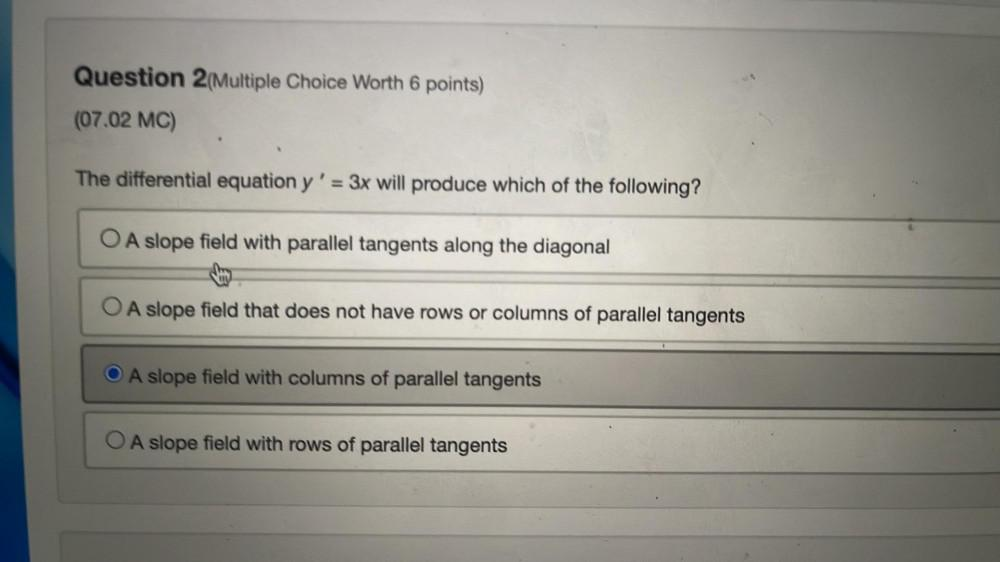Question:

# The differential equation y' = 3x will produce which of the

Last updated: 7/26/2022The differential equation y' = 3x will produce which of the following? A. A slope field with parallel tangents along the diagonal B. A slope field that does not have rows or columns of parallel tangents C. A slope field with columns of parallel tangents D. A slope field with rows of parallel tangents